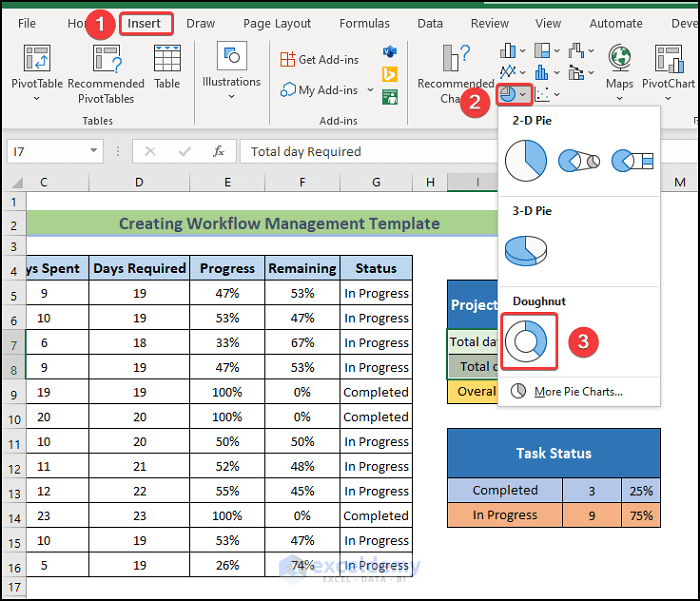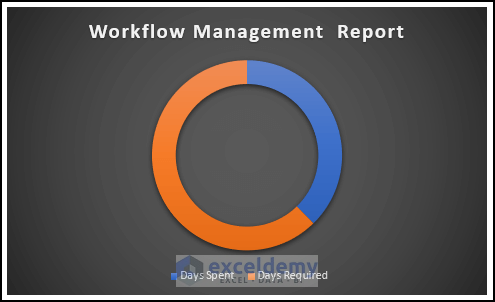# How to Create Workflow Management Template in Excel

Get FREE Advanced Excel Exercises with Solutions!

If you are looking for some special tricks to create a workflow management template in Excel, you’ve come to the right place. There is one way to create a workflow management template in Microsoft Excel. This article will discuss every step of this method in Excel. Let’s follow the complete guide to learn all of this.

## Workflow Management Template in Excel: Step-by-Step Procedure to Create

In the following section, we will use one effective and tricky method to create a workflow management template in Excel. This section provides extensive details on this method. You should learn and apply these to improve your thinking capability and Excel knowledge. We use the Microsoft Office 365 version here, but you can utilize any other version according to your preference.

### Step 1: Prepare Workplan Timeline

To create a workflow management template, we have to follow some specified steps. First, we want to prepare a work plan timeline. To do this, you have to follow the following things.

• Next, enter the Project name. Then, input the task name one by one.
• Now, input the responsible person’s name who is responsible for each task.
• After that, input the Starting Date of each task.
• Then, input the Due Date of each task.### Step 2: Create Workflow Layout in Excel

In this, we show how to create a workflow layout. Let’s walk through the following steps.

• Firstly, input the spent days by each task.
• Then, calculate the days required by using the following formula in cell H2.

`=F5-E5`

• Press Enter.• Next, drag the Fill handle icon.
• Therefore, you will get the Days Required column, as shown below.
• Then, calculate Progress by using the following formula in cell I2.

`=G5/H5`

• Press Enter.
• To convert the data into percentages, click on the Percentage.• Next, drag the Fill Handle icon.
• Therefore, you will get the Progress column, as shown below.• Then, input the progress status in the Status column.
• Finally, you will be able to get the following output.### Step 3: Build Tracker List

We want to make a template that takes off our loads to a great extent as we don’t have to do manual updates on certain calculations. Here, we will use the SUM, AVERAGE, and COUNTIF functions. To do this you have to follow the following things.

• At first, we have to copy some columns from the previous sheet by pressing ‘Ctrl+C’.
• Go to the new sheet, and press ‘Crl+V’ to paste it, as shown below.
• Next, in the remaining column, we have to use the following formula.

`=1-E5`

• Then, press Enter.• Next, drag the Fill Handle icon.
• Therefore, you will get the Remaining column, as shown below.• Afterward, to calculate the overall project completion, we have to use the following formula.

`=AVERAGE(E5:E16)`

The AVERAGE function returns the average of the twelve tasks, which is almost 60 percent.

• Next, press Enter.### Step 4: Create a Project Workflow Report

In this step, we will create a project workflow report. To do this task, follow the following process.

• First, to calculate the total days required, we have to use the following formula.

`=SUM(D5:D16)`

The SUM function returns the combined days of the Days Required column.

• Press Enter.• Next, to calculate the total days spent, we have to use the following formula.

`=SUM(C5:C16)`

The SUM function returns the combined days of the Days Spent column.

• Press Enter.• Now, to calculate the overall project progress, we have to use the following formula.

`=K8/K7`

• Press Enter.• Next, to create a dynamic summary like Task Status, we have to use the following formula.

`=COUNTIF(G5:G16,"Completed")`

The COUNTIF function will return the value by counting the number of cells of the range G5:G16, and the given condition is “Completed”. Using this approach, we will be able to calculate how many tasks have been completed in this project, which is a necessary step to track project workflow.

• Press Enter.• Next, we have to use the following formula in cell K14.

`=COUNTIF(G5:G16,"In Progress")`

The COUNTIF function will return the value by counting the number of cells of the range G5:G16, and the given condition is “In Progress”. Using this approach, we will be able to calculate how many tasks have been in progress in this project, which is a necessary step to track project workflow.

• Press Enter.• After that, to calculate the percentage of completed tasks, we have to use the following formula in cell L13.

`=K13/(K13+K14)`

• Press Enter.• To calculate the percentage of the In Progress tasks, we have to use the following formula in cell L14.

`=K14/(K14+K13)`

• Press Enter.
• Finally, we get the dynamic summary Task Status.### Step 5: Insert Excel Charts for Workflow Template

Now, we are going to create three different charts for the workflow management template. To do this, we have to follow the following steps.

• First, to create a chart, select the range of data and go to the Insert tab.  Next, select the Stacked Column chart.• Next, to modify the chart style, select Chart Design and then, select your desired Style 8 option from the Chart Styles group.
• As a consequence, you will get the following chart.• Afterward, to create a Pie Chart, select the range of data and go to the Insert tab.  Next, select the 3-D Pie chart.• As a consequence, you will get the following Pie chart.• To create a Doughnut chart, select the range of data and go to the Insert tab. Next, select the Doughnut chart.

###• As a consequence, you will get the following Doughnut chart.

###Read More: How to Create Workflow Chart in Excel

### Step 6: Generate Workflow Management Summary

Now, we will create a workflow management summary. To do this, we are going to show our charts in a new sheet as a report.

• To create a dynamic workflow management summary, first, you have to create a new sheet and set the name of that sheet as Final Output.
• Next, you have to copy every chart by pressing ‘Ctrl+C’ to go to the Final Output sheet, and press ‘Crl+V’ to paste it.
• As a consequence, you will get the final output like the following.Download this practice workbook to exercise while you are reading this article. It contains all the datasets in different spreadsheets for a clear understanding. Try it yourself while you go through the step-by-step process.

## Conclusion

That’s the end of today’s session. I strongly believe that from now you may be able to create a workflow management template in Excel. If you have any queries or recommendations, please share them in the comments section below.

## What is ExcelDemy?

ExcelDemy Learn Excel & Excel Solutions Center provides free Excel tutorials, free support , online Excel training and Excel consultancy services for Excel professionals and businesses. Feel free to contact us with your Excel problems.Welcome to my Profile. I am working on and researching Microsoft Excel right now, and I will be posting articles about it here. I received a B.Sc. in Naval Architecture and Marine Engineering from the Bangladesh University of Engineering and Technology (BUET). Having studied naval architecture, I have a strong interest in research and development. Always try to learn from different sources and come up with creative solutions.

We will be happy to hear your thoughtsAdvanced Excel Exercises with Solutions PDF